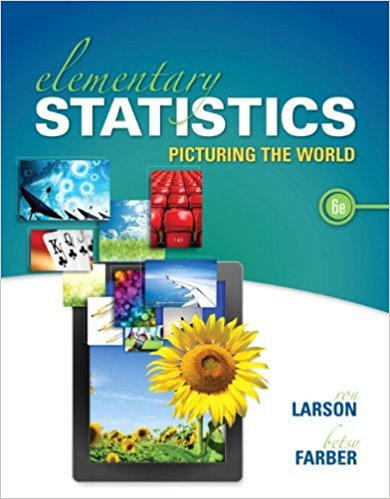×
×

# Solutions for Chapter 9.4: Multiple Regression## Full solutions for Elementary Statistics: Picturing the World | 6th Edition

ISBN: 9780321911216Solutions for Chapter 9.4: Multiple Regression

Solutions for Chapter 9.4
4 5 0 381 Reviews
27
2
##### ISBN: 9780321911216

Elementary Statistics: Picturing the World was written by and is associated to the ISBN: 9780321911216. Chapter 9.4: Multiple Regression includes 6 full step-by-step solutions. This expansive textbook survival guide covers the following chapters and their solutions. This textbook survival guide was created for the textbook: Elementary Statistics: Picturing the World , edition: 6. Since 6 problems in chapter 9.4: Multiple Regression have been answered, more than 98501 students have viewed full step-by-step solutions from this chapter.

Key Statistics Terms and definitions covered in this textbook
• Asymptotic relative eficiency (ARE)

Used to compare hypothesis tests. The ARE of one test relative to another is the limiting ratio of the sample sizes necessary to obtain identical error probabilities for the two procedures.

• Average run length, or ARL

The average number of samples taken in a process monitoring or inspection scheme until the scheme signals that the process is operating at a level different from the level in which it began.

• Chance cause

The portion of the variability in a set of observations that is due to only random forces and which cannot be traced to speciic sources, such as operators, materials, or equipment. Also called a common cause.

• Chi-square test

Any test of signiicance based on the chi-square distribution. The most common chi-square tests are (1) testing hypotheses about the variance or standard deviation of a normal distribution and (2) testing goodness of it of a theoretical distribution to sample data

• Completely randomized design (or experiment)

A type of experimental design in which the treatments or design factors are assigned to the experimental units in a random manner. In designed experiments, a completely randomized design results from running all of the treatment combinations in random order.

• Conditional probability mass function

The probability mass function of the conditional probability distribution of a discrete random variable.

• Continuous distribution

A probability distribution for a continuous random variable.

• Cook’s distance

In regression, Cook’s distance is a measure of the inluence of each individual observation on the estimates of the regression model parameters. It expresses the distance that the vector of model parameter estimates with the ith observation removed lies from the vector of model parameter estimates based on all observations. Large values of Cook’s distance indicate that the observation is inluential.

• Counting techniques

Formulas used to determine the number of elements in sample spaces and events.

• Crossed factors

Another name for factors that are arranged in a factorial experiment.

• Density function

Another name for a probability density function

• Eficiency

A concept in parameter estimation that uses the variances of different estimators; essentially, an estimator is more eficient than another estimator if it has smaller variance. When estimators are biased, the concept requires modiication.

• Estimator (or point estimator)

A procedure for producing an estimate of a parameter of interest. An estimator is usually a function of only sample data values, and when these data values are available, it results in an estimate of the parameter of interest.

• Event

A subset of a sample space.

• Extra sum of squares method

A method used in regression analysis to conduct a hypothesis test for the additional contribution of one or more variables to a model.

• F-test

Any test of signiicance involving the F distribution. The most common F-tests are (1) testing hypotheses about the variances or standard deviations of two independent normal distributions, (2) testing hypotheses about treatment means or variance components in the analysis of variance, and (3) testing signiicance of regression or tests on subsets of parameters in a regression model.

• Forward selection

A method of variable selection in regression, where variables are inserted one at a time into the model until no other variables that contribute signiicantly to the model can be found.

• Fractional factorial experiment

A type of factorial experiment in which not all possible treatment combinations are run. This is usually done to reduce the size of an experiment with several factors.

• Gamma function

A function used in the probability density function of a gamma random variable that can be considered to extend factorials

• Gamma random variable

A random variable that generalizes an Erlang random variable to noninteger values of the parameter r

×